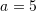### Corrosion

### Corrosion:

The error after iteration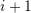is equal to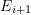.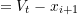while that in iterationis equal to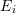.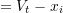. Therefore, the above expression yields:

Background information. Any other information………….
Cathodic Protection

### Corrosion Growth Rate:

What is it? How to calculate it. (The pipeline integrity corrosion growth rate plan document can be summarized here)
[simple_table cols=”CGR PE Tape,CGR all other coating, RPR CGR PE Tape coating,RPR CGR all other coating” data=”0.11,0.08,0.01,0.008″] Examples:
Historical based assumptions
[
C=FOStimesfrac {d_{ILI}}{Y_{ILI}-Y_{Construction}}
] Where: (C) is the corrosion growth rate, (FOS) is the required factor of safety, (d_{ILI}) is the ILI measured depth of corrosion, (Y_{ILI}) is the year the corrosion was measured, and
(Y_{Construction}) is the year of the construction of the line. The above equation assumes a linear corrosion growth and that corrosion started at the year of construction.
Example:

### Corrosion Assessment:

How to assess corrosion………

What is RPR…..

Examples…

### Corrosion Assessment Decision:

Line 1.. line 2… line 3..
Decision are made and input into a database. Field data should be input as well

### Historical Corrosion Assessment:

Line 1.. line 2… line 3..
Preview of corrosion assessments database per line.

This is latex within a paragraph